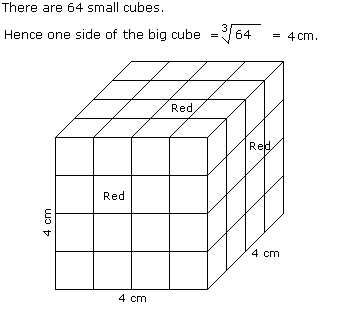# Verbal Reasoning - Cube and Cuboid - Discussion

The following questions are based on the information given below:

1. All the faces of cubes are painted with red colour.
2. The cubes is cut into 64 equal small cubes.

1.

How many small cubes have only one face coloured ?

 [A]. 4 [B]. 8 [C]. 16 [D]. 24

Explanation:Number of small cubes having only one face coloured = (x - 2)2 x No. of faces

= (4 - 2)2 x 6

= 24